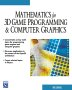# Maths - Quadratic Form

A quadratic form is an expression in a number of variables where each term is of degree two.

For instance, if there are two variables: x and y, then the terms will contain:

x² ,xy or y²

possibly multiplied by some constant.

## Representing a Quadratic Form by a Symmetric Matrix

Every quadratic form can be represented by a symmetric matrix, to generate the quadratic form we pre-multiply the matrix by a single row vector containing the variables and post-multiply the matrix by a single column vector also containing the variables.

Here is a two dimensional example:

 x y
 1 3 3 1
 x y
=x² + 6xy + y²

and here is a three dimensional example:

 x y z
 0 1 1 1 3 5 1 5 0
 x y z
= 3y² + 2xy + 2xz +10yz

## Applications of Quadratic Form

On this page we use it to define the dot product.

### Representing spaces

There is a relationship between the quadratic form, the dot product (or more generally the symmetric bilinear form) and the metric of a space. For instance, if we have a two dimensional Euclidean space, where a given point is represented by the [x,y] coordinate then the distance from the origin is given by the square root of:

 x y
 1 0 0 1
 x y
= x² + y²

Or, equivalently, if we we let the vector: v= [x,y] then the distance from the origin is given by the square root of:

v•v =x² + y²

We can also represent other types of space, for instance, a Minkowski type space could be represented by:

 x y
 1 0 0 -1
 x y
= x² - y²

Other matrices can be used, where the non-diagonal terms are not zero, although since the matrix is symmetric it is diagonalizable. So there will exist a new "basis" (i.e. new coordinate system) in which the matrix is diagonal. Those give the "principle directions" for the surface defined by the quadratic form.

## Bilinear Form

The quadratic form is a bilinear form (described on this page) where the multiplication is commutative.

## Program

There are a number of open source programs that can allow us to work with quadratic form. I have used Axiom, how to install Axiom here.

We can work purely with matrix values:

I have put user input in red:

 ```(1) -> [[x,y]]*[[1,3],[3,1]]*[[x],[y]] (1) + 2 2+ +y + 6x y + x + Type: Matrix Polynomial Integer ```

Or there is a specific quadratic form type:

 `(2) -> quadraticForm(matrix[[1,3],[3,1]]) +1 3+ (2) | | +3 1+ `

## Applications

Some topics which use quadratic form are:

 metadata block see also: Correspondence about this page Book Shop - Further reading. Where I can, I have put links to Amazon for books that are relevant to the subject, click on the appropriate country flag to get more details of the book or to buy it from them.Mathematics for 3D game Programming - Includes introduction to Vectors, Matrices, Transforms and Trigonometry. (But no euler angles or quaternions). Also includes ray tracing and some linear & rotational physics also collision detection (but not collision response). Other Math Books Specific to this page here:

This site may have errors. Don't use for critical systems.